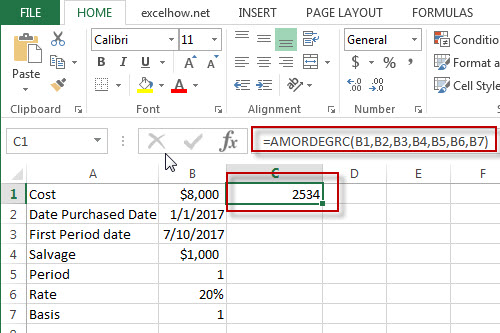# Excel Amordegrc Function

This post will guide you how to use Excel AMORDEGRC function with syntax and examples in Microsoft excel.

### Description

The Excel AMORDEGRC function returns the linear depreciation of an asset for each accounting period by using a depreciation coefficient.

The AMORDEGRC function is a build-in function in Microsoft Excel and it is categorized as a Financial Function.

The AMORDEGRC function is available in Excel 2016, Excel 2013, Excel 2010, Excel 2007, Excel 2011 for Mac.

### Syntax

The syntax of the AMORDEGRC function is as below:

`= AMORDEGRC (cost, date_purchased, first_period, salvage, period, rate, [basis])`

Where the AMORDEGRC function arguments are:

• Cost -This is a required argument. The cost of the asset.
• Date_purchased – This is a required argument.  The date that the asset was purchased.
• First_period – This is a required argument.  The date of the end of the first period.
• Salvage – This is a required argument.  The salvage value at the end of the life of the asset.
• Period – This is a required argument.  The period in which to calculate the depreciation.
• Rate– This is a required argument.  The rate of depreciation.
• Basis – This is a required argument.  The year basis to be used. The following values can be used:
 Basis Date system 0 or omitted 360 days (NASD method) 1 Actual 3 365 days in a year 4 360 days in a year (European method)

### Excel AMORDEGRC Function Examples

The below examples will show you how to use Excel AMORDEGRC Function to calculate the linear depreciation of an asset for each accounting period.

#1 =AMORDEGRC(B1,B2,B3,B4,B5,B6,B7)### Related Functions

• Excel Amorlinc Function
The Excel AMORLINC function returns the linear depreciation of an asset for each accounting period. This function is provided for French accounting system.The syntax of the AMORLINC function is as below:= AMORLINC (cost, date_purchased, first_period, salvage, period, rate, [basis])…
Related Posts

Excel DVARP Function

This post will guide you how to use Excel DVARP function with syntax and examples in Microsoft excel. Description The Excel DVARP Function will get the variance of a population based on the entire population of numbers in a column ...

Excel DVAR Function

This post will guide you how to use Excel DVAR function with syntax and examples in Microsoft excel. Description The Excel DVAR Function will get the variance of a population based on a sample of numbers in a column in ...

Excel DSUM Function

This post will guide you how to use Excel DSUM function with syntax and examples in Microsoft excel. Description The Excel DSUM Function will add the numbers in a column or database that meets a given criteria. And so you ...

Excel DSTDEVP Function

This post will guide you how to use Excel DSTDEVP function with syntax and examples in Microsoft excel. Description The Excel DSTDEVP returns the standard deviation of a population based on the entire population of numbers in a column or ...

Excel DSTDEV Function

This post will guide you how to use Excel DSTDEV function with syntax and examples in Microsoft excel. Description The Excel DSTDEV returns the standard deviation of a population based on a sample of numbers in a column or database ...

Excel DMIN Function

This post will guide you how to use Excel DMIN function with syntax and examples in Microsoft excel. Description The Excel DMIN returns the minimum value from a database or a column of a list that matches the specified conditions. ...

Excel DPRODUCT Function

This post will guide you how to use Excel DPRODUCT function with syntax and examples in Microsoft excel. Description The Excel DPRODUCT returns the product of values from a set of records that match criteria that you specify. The DPRODUCT ...

Excel XIRR Function

This post will guide you how to use Excel XIRR function with syntax and examples in Microsoft excel. Description The Excel XIRR function returns the internal rate of return for a series of cash flows that is not necessarily periodic. ...

Excel VDB Function

This post will guide you how to use Excel VDB function with syntax and examples in Microsoft excel. Description The Excel VDB function calculates the depreciation of an asset for a specified period based on the double declining balance method. ...

Excel SYD Function

This post will guide you how to use Excel SYD function with syntax and examples in Microsoft excel. Description The Excel SYD function calculates the sum-of-years’digits depreciation of an asset for a specified period. And it will return a numeric ...

Sidebar